Next: Evection Up: Lunar motion Previous: Annual equation

# Parallactic inequality

Next, let us consider terms in the solution of the lunar equations of motions that depend linearly on the ratio of the major radii of the lunar and the solar orbits,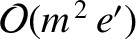.

According to Equations (11.138) and (11.150),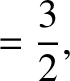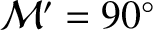(11.246) and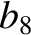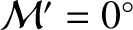(11.247)

where we have neglected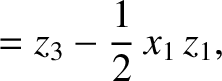contributions. It follows from Equations (11.192), (11.193), and (11.204) that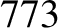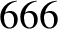(11.248) and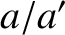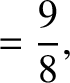(11.249)

To next order in, Equations (11.138) and (11.150) give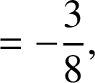(11.250) and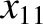(11.251)

where use has been made of Equations (11.217) and (11.218), as well as the previous expressions for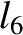and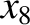. Hence, Equations (11.192), (11.193), and (11.204) yield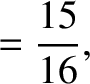(11.252) and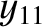(11.253)

According to Equations (11.139) and (11.151),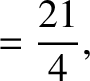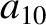(11.254) and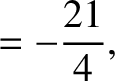(11.255)

Equations (11.192), (11.193), and (11.205) yield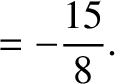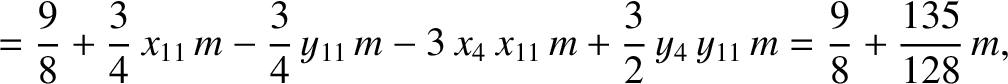(11.256) and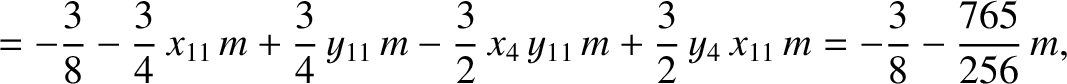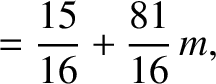(11.257)

It follows from Equations (11.76), (11.122)-(11.124), (11.170), (11.171), (11.182), and (11.183), as well as the previous expressions for,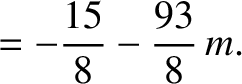,, and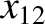, that the net perturbation to the lunar orbit due to terms in the solution of the lunar equations of motion that depend linearly onis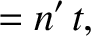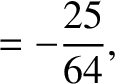(11.258)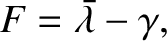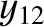(11.259) and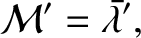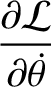(11.260)

Here,is the mass of the Earth, and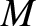the mass of the moon. The previous expressions are accurate to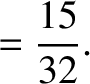.

The first term on the right-hand side of Equation (11.259) is known as the parallactic inequality. The parallactic inequality attains its maximum amplitude when the Moon in half illuminated (i.e., whenor). Conversely, the amplitude of the parallactic inequality is zero when the Moon is either fully illuminated or not illuminated at all (i.e., when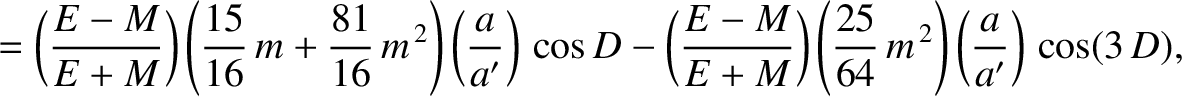or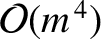). According to Equation (11.259), the parallactic inequality generates a perturbation in the lunar ecliptic longitude that oscillates with a period of a synodic month, and has an amplitude (calculated using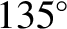,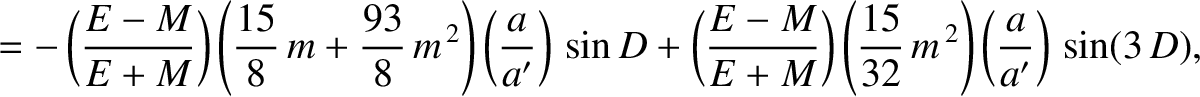, and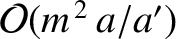) of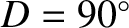arc seconds (Yoder 1995). As before, the oscillation period is in good agreement with observations, whereas the amplitude is somewhat inaccurate [it should be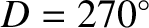arc seconds (Chapront-Touzé and Chapront 1988)] because of the omission of higher-order (in) contributions.Next: Evection Up: Lunar motion Previous: Annual equation
Richard Fitzpatrick 2016-03-31